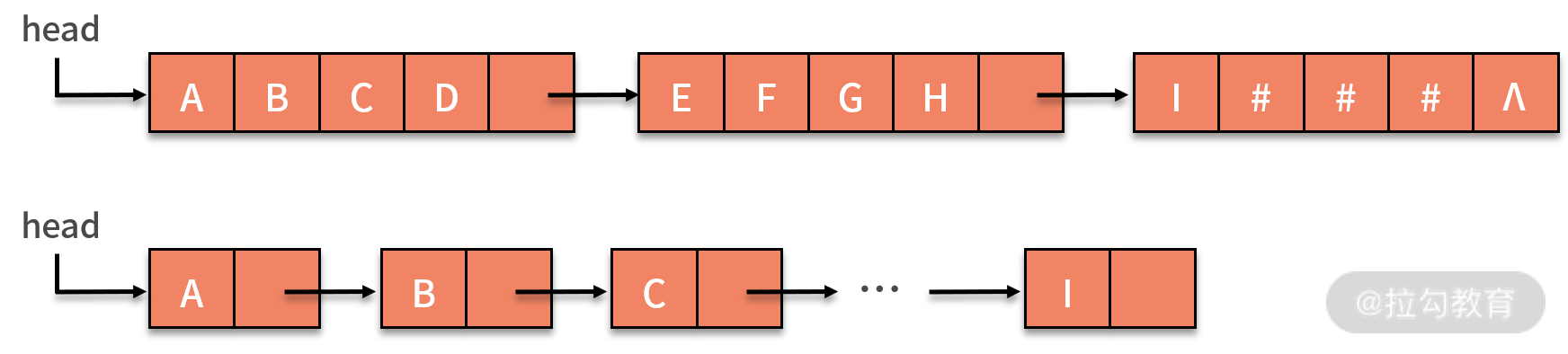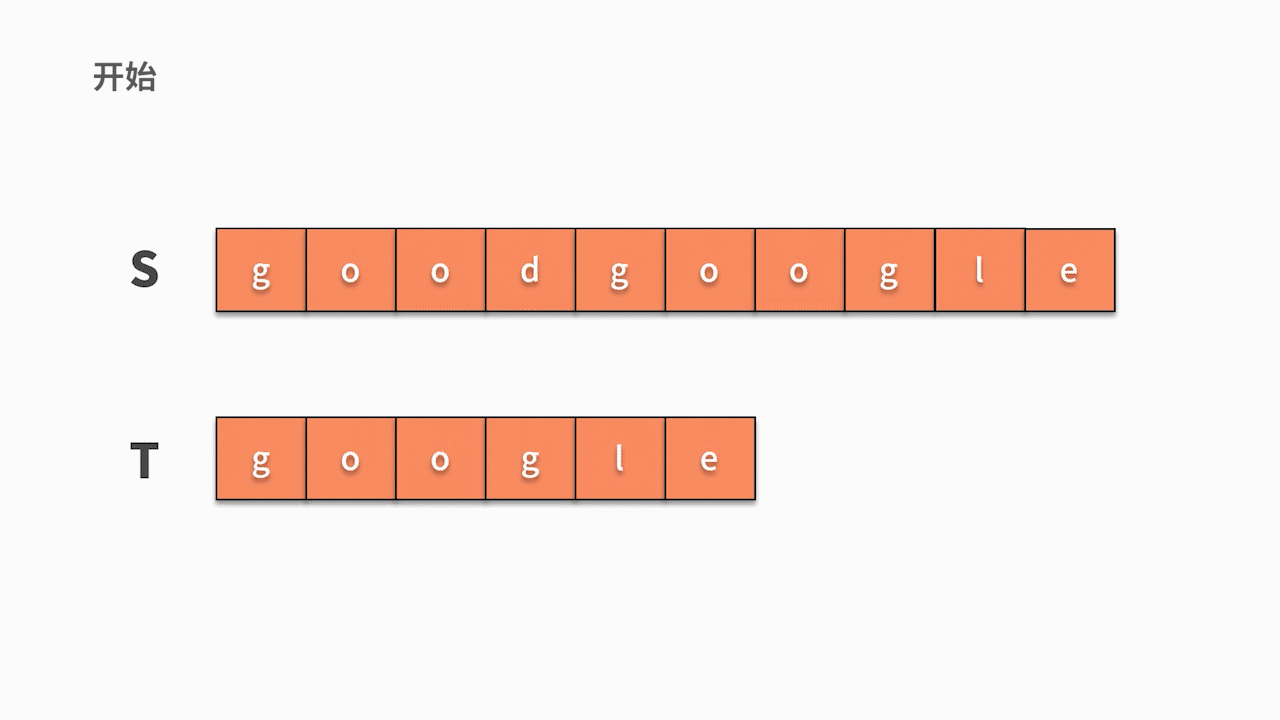08 字符串：如何正确回答面试中高频考察的字符串匹配算法？

字符串是什么

• 空串，指含有零个字符的串。例如，s = ""，书面中也可以直接用 Ø 表示。
• 空格串，只包含空格的串。它和空串是不一样的，空格串中是有内容的，只不过包含的是空格，且空格串中可以包含多个空格。例如，s = " "，就是包含了 3 个空格的字符串。
• 子串，串中任意连续字符组成的字符串叫作该串的子串。
• 原串通常也称为主串。例如：a = "BEI"，b = "BEIJING"，c = "BJINGEI" 。
• 对于字符串 a 和 b 来说，由于 b 中含有字符串 a ，所以可以称 a 是 b 的子串，b 是 a 的主串；
• 而对于 c 和 a 而言，虽然 c 中也含有 a 的全部字符，但不是连续的 "BEI" ，所以串 c 和 a 没有任何关系。

• 字符串的顺序存储结构，是用一组地址连续的存储单元来存储串中的字符序列，一般是用定长数组来实现。有些语言会在串值后面加一个不计入串长度的结束标记符，比如 \0 来表示串值的终结。
• 字符串的链式存储结构，与线性表是相似的，但由于串结构的特殊性（结构中的每个元素数据都是一个字符），如果也简单地将每个链结点存储为一个字符，就会造成很大的空间浪费。因此，一个结点可以考虑存放多个字符，如果最后一个结点未被占满时，可以使用 "#" 或其他非串值字符补全，如下图所示：• 如果字符串中包含的数据量很大，但是可用的存储空间有限，那么就需要提高空间利用率，相应地减少结点数量。
• 而如果程序中需要大量地插入或者删除数据，如果每个节点包含的字符过多，操作字符就会变得很麻烦，为实现功能增加了障碍。

字符串的基本操作

子串查找（字符串匹配）

• 首先，我们从主串 s 第 1 位开始，判断 s 的第 1 个字符是否与 t 的第 1 个字符相等。
• 如果不相等，则继续判断主串的第 2 个字符是否与 t 的第1 个字符相等。直到在 s 中找到与 t 第一个字符相等的字符时，然后开始判断它之后的字符是否仍然与 t 的后续字符相等。
• 如果持续相等直到 t 的最后一个字符，则匹配成功。
• 如果发现一个不等的字符，则重新回到前面的步骤中，查找 s 中是否有字符与 t 的第一个字符相等。
• 如下图所示，s 的第1 个字符和 t 的第 1 个字符相等，则开始匹配后续。直到发现前三个字母都匹配成功，但 s 的第 4 个字母匹配失败，则回到主串继续寻找和 t 的第一个字符相等的字符。
• 如下图所示，这时我们发现主串 s 第 5 位开始相等，并且随后的 6 个字母全匹配成功，则找到结果。public void s1() {
int isfind = 0;

for (int i = 0; i < s.length() - t.length() + 1; i++) {
if (s.charAt(i) == t.charAt(0)) {
int jc = 0;
for (int j = 0; j < t.length(); j++) {
if (s.charAt(i + j) != t.charAt(j)) {
break;
}
jc = j;
}
if (jc == t.length() - 1) {
isfind = 1;
}
}
}
System.out.println(isfind);
}

字符串匹配算法的案例

• 首先，你需要对于字符串 a 和 b 找到第一个共同出现的字符，这跟前面讲到的匹配算法在主串中查找第一个模式串字符一样。
• 然后，一旦找到了第一个匹配的字符之后，就可以同时在 a 和 b 中继续匹配它后续的字符是否相等。这样 a 和 b 中每个互相匹配的字串都会被访问一遍。全局还要维护一个最长子串及其长度的变量，就可以完成了。

public void s2() {
String a = "123456";
String b = "13452439";
String maxSubStr = "";
int max_len = 0;

for (int i = 0; i < a.length(); i++) {
for (int j = 0; j < b.length(); j++){
if (a.charAt(i) == b.charAt(j)){
for (int m=i, n=j; m<a.length()&&n<b.length(); m++,n++) {
if (a.charAt(m) != b.charAt(n)){
break;
}
if (max_len < m-i+1){
max_len = m-i+1;
maxSubStr = a.substring(i, m+1);
}
}
}
}
}
System.out.println(maxSubStr);
}

总结

• 在线性表的基本操作中，大多以“单个元素”作为操作对象；
• 在字符串的基本操作中，通常以“串的整体”作为操作对象；
• 字符串的增删操作和数组很像，复杂度也与之一样。但字符串的查找操作就复杂多了，它是参加面试、笔试常常被考察的内容。